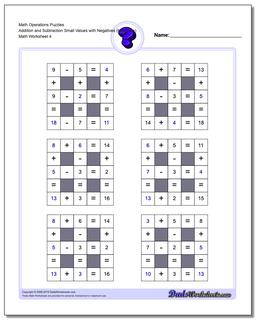# Math Worksheets: Number Grid Puzzles: Number Grid Puzzles: Math Operations Puzzles Addition and Subtraction Small Values with Negatives (Moderate) (Fourth Worksheet)## Math Operations Puzzles Addition and Subtraction Small Values with Negatives (Moderate) (Fourth Worksheet)

PropertyValue
DescriptionMath Operations Puzzles Addition and Subtraction Small Values with Negatives (Moderate): Math puzzle worksheets that require students to fill in missing numbers with smaller numbers. (Fourth Worksheet)
Resource TypeWorksheet